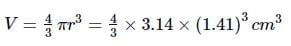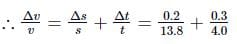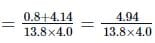NEET  >  Test: Significant Figures (NCERT)

# Test: Significant Figures (NCERT) - NEET

Test Description

## 10 Questions MCQ Test Physics Class 11 - Test: Significant Figures (NCERT)

Test: Significant Figures (NCERT) for NEET 2023 is part of Physics Class 11 preparation. The Test: Significant Figures (NCERT) questions and answers have been prepared according to the NEET exam syllabus.The Test: Significant Figures (NCERT) MCQs are made for NEET 2023 Exam. Find important definitions, questions, notes, meanings, examples, exercises, MCQs and online tests for Test: Significant Figures (NCERT) below.
Solutions of Test: Significant Figures (NCERT) questions in English are available as part of our Physics Class 11 for NEET & Test: Significant Figures (NCERT) solutions in Hindi for Physics Class 11 course. Download more important topics, notes, lectures and mock test series for NEET Exam by signing up for free. Attempt Test: Significant Figures (NCERT) | 10 questions in 10 minutes | Mock test for NEET preparation | Free important questions MCQ to study Physics Class 11 for NEET Exam | Download free PDF with solutions
 1 Crore+ students have signed up on EduRev. Have you?
Test: Significant Figures (NCERT) - Question 1

### Which of the following statements is incorrect regarding significant figures?

Detailed Solution for Test: Significant Figures (NCERT) - Question 1

The power of 10 is irrelevant to the determination of significant figures.

Test: Significant Figures (NCERT) - Question 2

### The value of resistance is 10.845 Ω and the current is 3.23 A. On multiplying, we get the potential difference is 35.02935 V. The value of potential difference in terms of significant figures would be

Detailed Solution for Test: Significant Figures (NCERT) - Question 2

The final result should be 3 significant figures.

Test: Significant Figures (NCERT) - Question 3

### A cube has a side of length 1.2 x 10-2m. Its volume upto correct significant figures is

Detailed Solution for Test: Significant Figures (NCERT) - Question 3

Here
Length of the cube, L = 1.2 x 10-2 m
Volume of the cube, V = (1.2 x 10-2m)3 = 1.728 x 10-6 m3
As the result can have only two significant figures, therefore, on rounding off, we get, V = 1.7 x 10-6 m3

Test: Significant Figures (NCERT) - Question 4

The radius of a sphere is 1.41cm. Its volume to an appropriate number of significant figures is

Detailed Solution for Test: Significant Figures (NCERT) - Question 4

Radius of the sphere, r = 1.41cm
(3 significant figures)
Volume of the sphere,= 11.736 cm3
Rounded off upto 3 significant figures = 11.7 cm3.

Test: Significant Figures (NCERT) - Question 5

The mass of a box measured by a grocer's balance is 2.3 kg. Two gold pieces of masses 20.15 g and 20.17 g are added to the box. The total mass of the box is:

Detailed Solution for Test: Significant Figures (NCERT) - Question 5

Here, mass of the box, m = 2.3 kg
Mass of one gold piece, m1 = 20.15 g = 0.02015 kg
Mass of other gold piece, m2 = 20.17 g = 0.02017 kg
∴ Total mass = m + m1 + m2
= 2.3 kg + 0.02015 kg + 0.02017 kg = 2.34032 kg
As the result is correct only upto one place of decimal, therefore, on rounding off, we get
Total mass = 2.3 kg

Test: Significant Figures (NCERT) - Question 6

The mass of a box measured by a grocer's balance is 2.3 kg. Two gold pieces of masses 20.15 g and 20.17 g are added to the box.

What is the difference in the masses of the pieces?

Detailed Solution for Test: Significant Figures (NCERT) - Question 6

Difference in masses
= m2 - m1 = 20.17 g - 20.15 g
= 0.02 g (correct upto two places of decimal)

Test: Significant Figures (NCERT) - Question 7

The numbers 3.845 and 3.835 on rounding off to 3 significant figures will give

Detailed Solution for Test: Significant Figures (NCERT) - Question 7

The number 3.845 rounded off to three significant figures becomes 3.84 since the preceding digit is even. On the other hand, the number 3.835 rounded off to three significant figures becomes 3.84 since the preceding digit is odd.

Test: Significant Figures (NCERT) - Question 8

The respective number of significant figures for the numbers 6.320, 6.032, 0.0006032 are

Detailed Solution for Test: Significant Figures (NCERT) - Question 8

According to the rules of significant figures 6.320 has four significant figures.
6.032 has four significant figures. 0.0006032 has four significant figures.

Test: Significant Figures (NCERT) - Question 9

The number of significant figures in the numbers 4.8000 x 104 and 48000.50 are respectively

Detailed Solution for Test: Significant Figures (NCERT) - Question 9

As per rule of significant figures, 4.8000 x 104 has 5 significant figures and 48000.50 has 7 significant figures.

Test: Significant Figures (NCERT) - Question 10

A body travels uniformly a distance of (13.8 ± 0.2) m in a time (4.0 ± 0.3) s. Its velocity with error limits is

Detailed Solution for Test: Significant Figures (NCERT) - Question 10

Here, s = (13.8 ± 0.2) m, t = (4.0 ± 0.3)s
∴ veliocity, v = s/t = 13.8/4.0 = 3.45 m s−1
(Rounded off to first place of decimal)= 0.0865
or Δv = v × 0.0865 = 3.45 × 0.0865
= 0.3087.
∴ velocity =(3.5 ± 0.3) m s−1.

## Physics Class 11

130 videos|483 docs|210 tests
Information about Test: Significant Figures (NCERT) Page
In this test you can find the Exam questions for Test: Significant Figures (NCERT) solved & explained in the simplest way possible. Besides giving Questions and answers for Test: Significant Figures (NCERT), EduRev gives you an ample number of Online tests for practice

## Physics Class 11

130 videos|483 docs|210 tests

### How to Prepare for NEET

Read our guide to prepare for NEET which is created by Toppers & the best Teachers(Scan QR code)